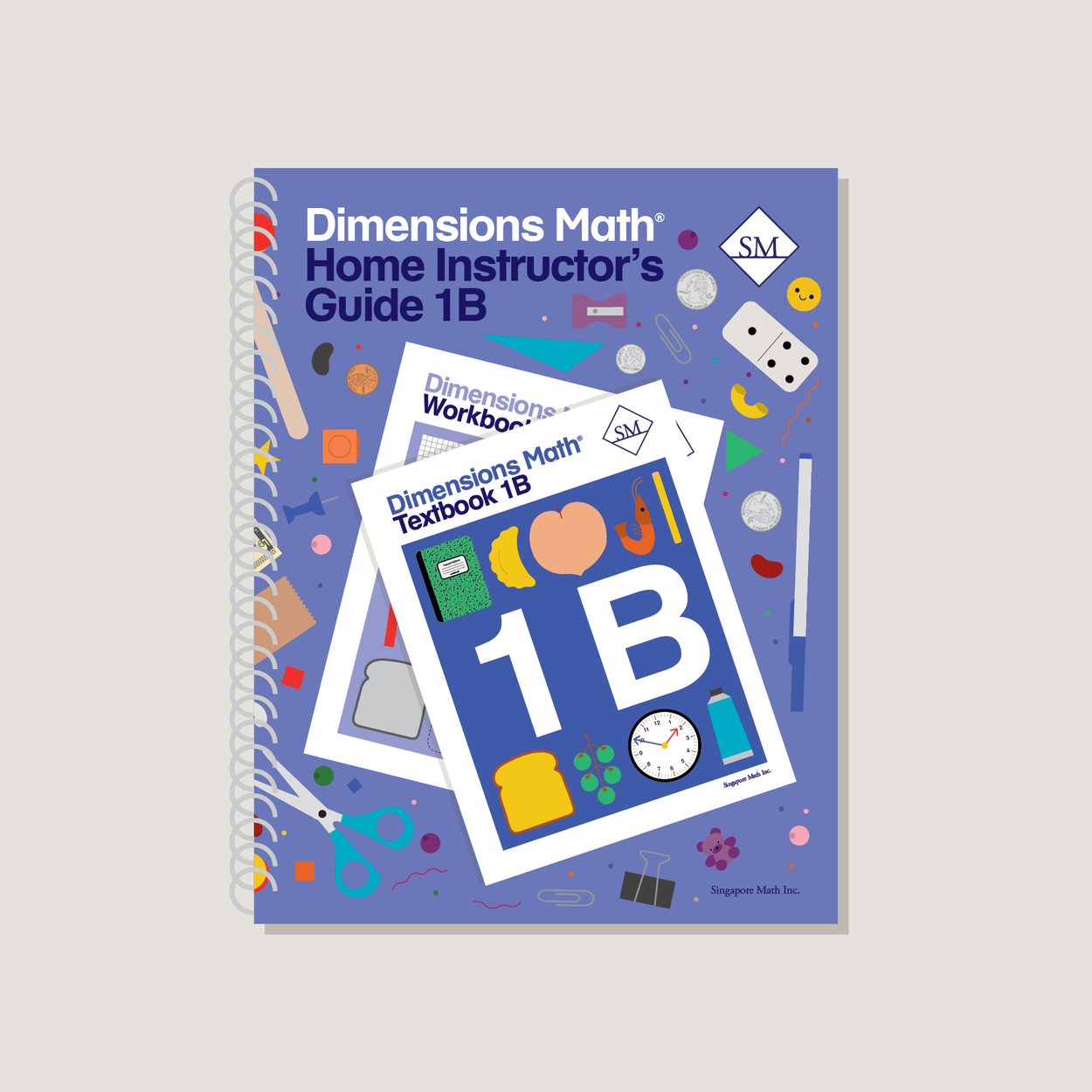Dimensions Math Home Instructor's Guide 1B

\$20.00

Dimensions Math Home Instructor’s Guides are an essential resource for teaching Dimensions Math in a home or one-on-one setting. The purpose of the Guides is to help educators understand concepts in the Dimensions Math curriculum and teach as effectively as possible. They include extensive background notes, lesson suggestions, tips, and activities. Home Instructor’s Guides include answer keys for corresponding Dimensions Math Textbooks and Workbooks.

Note: Soft cover, spiral bound.

SKU: DMHIG1B

ISBN: 9781947226593

Pagecount: 152

Dimensions: 8.5 x 11 x 0.75 in

Weight: 1.2 lbs

Binding: Spiral

Color: Grayscale

Cover: Soft

Perforated: No

Sample Pages

Chapter 10: Length
Lesson 1: Comparing Lengths Directly
Lesson 2: Comparing Lengths Indirectly
Lesson 3: Comparing Lengths with Units
Lesson 4: Practice

Chapter 11: Comparing
Lesson 1: Subtraction as Comparison
Lesson 2: Making Comparison Subtraction Stories
Lesson 3: Picture Graphs
Lesson 4: Practice

Chapter 12: Numbers to 40
Lesson 1: Numbers to 40
Lesson 2: Tens and Ones
Lesson 3: Counting by Tens and Ones
Lesson 4: Comparing
Lesson 5: Practice

Chapter 13: Addition and Subtraction Within 40
Lesson 2: Subtract Ones
Lesson 3: Make the Next Ten
Lesson 5: Subtract from Tens
Lesson 6: Use Subtraction Facts
Lesson 8: Practice

Chapter 14: Grouping and Sharing
Lesson 2: Sharing
Lesson 3: Grouping
Lesson 4: Practice

Chapter 15: Fractions
Lesson 1: Halves
Lesson 2: Fourths
Lesson 3: Practice
Review 3

Chapter 16: Numbers to 100
Lesson 1: Numbers to 100
Lesson 2: Tens and Ones
Lesson 3: Count by Ones or Tens
Lesson 4: Compare Numbers to 100
Lesson 5: Practice

Chapter 17: Addition and Subtraction Within 100
Lesson 1: Add Ones — Part 1
Lesson 3: Add Ones — Part 2
Lesson 4: Add Tens and Ones — Part 1
Lesson 5: Add Tens and Ones — Part 2
Lesson 6: Subtract Ones — Part 1
Lesson 7: Subtract from Tens
Lesson 8: Subtract Ones — Part 2
Lesson 9: Subtract Tens
Lesson 10: Subtract Tens and Ones — Part 1
Lesson 11: Subtract Tens and Ones — Part 2
Lesson 12: Practice

Chapter 18: Time
Lesson 1: Telling Time to the Hour
Lesson 2: Telling Time to the Half Hour
Lesson 3: Telling Time to the 5 Minutes
Lesson 4: Practice

Chapter 19: Money
Lesson 1: Coins
Lesson 2: Counting Money
Lesson 3: Bills
Lesson 4: Shopping
Lesson 5: Practice
Review 4

A & B Books: Our programs divide the school year into two semesters. “A” level books are for the first half of the school year. “B” level books are for the second half of the school year. You need both “A” and “B” material for a complete school year.

Required Components: Textbooks, Workbooks, and Guides (either Home Instructor’s Guides or Teacher’s Guides) are all necessary components. These three elements each serve a unique function and work together to build math mastery.

Printouts: Dimensions Math PK-5 Resources

Recommended Manipulatives: Dimensions Math Grades PK-5 Recommended Manipulatives